Under steady-state condition the GREEN's functions depend on time differences. One usually FOURIER transforms the time difference coordinate,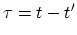, to energy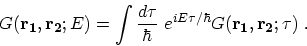(3.70)

Under steady-state condition the quantum kinetic equations, (3.64), (3.65), and (3.69), can be written as :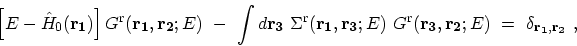(3.71)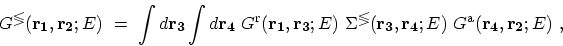(3.72)

where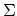is the total self-energy. A similar transformation can be applied to self-energies. However, to obtain self-energies one has to first apply LANGRETH's rules and then FOURIER transform the time difference coordinate to energy. We consider the self-energies discussed in Section 3.6. The evaluation of the HARTREE self-energy due to electron-electron interaction is straightforward, since it only includes the electron GREEN's function. However, the lowest-order self-energy due to electron-phonon interaction contains the products of the electron and phonon GREEN's functions. Using LANGRETH's rules (Table 3.1) and then FOURIER transforming the self-energies due to electron-phonon interaction, (3.50) takes the form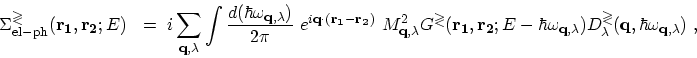(3.73)

To calculate the retarded self-energy, however, it is more straightforward to FOURIER transform the relation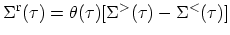, see (3.52). By defining the broadening function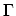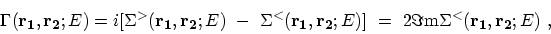(3.74)

the retarded self-energy is given by the convolution of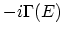and the FOURIER transform of the step function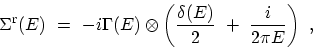(3.75)

where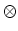denotes the convolution. Therefore, the retarded self-energy is given by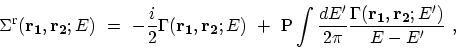(3.76)

where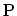stands for principal part.

M. Pourfath: Numerical Study of Quantum Transport in Carbon Nanotube-Based Transistors